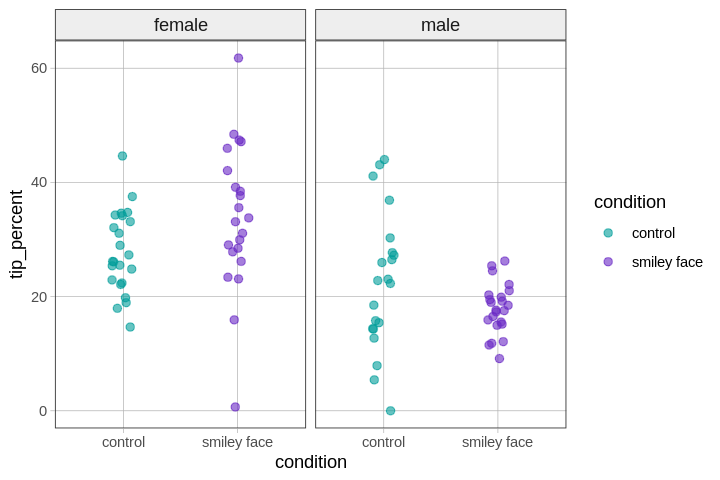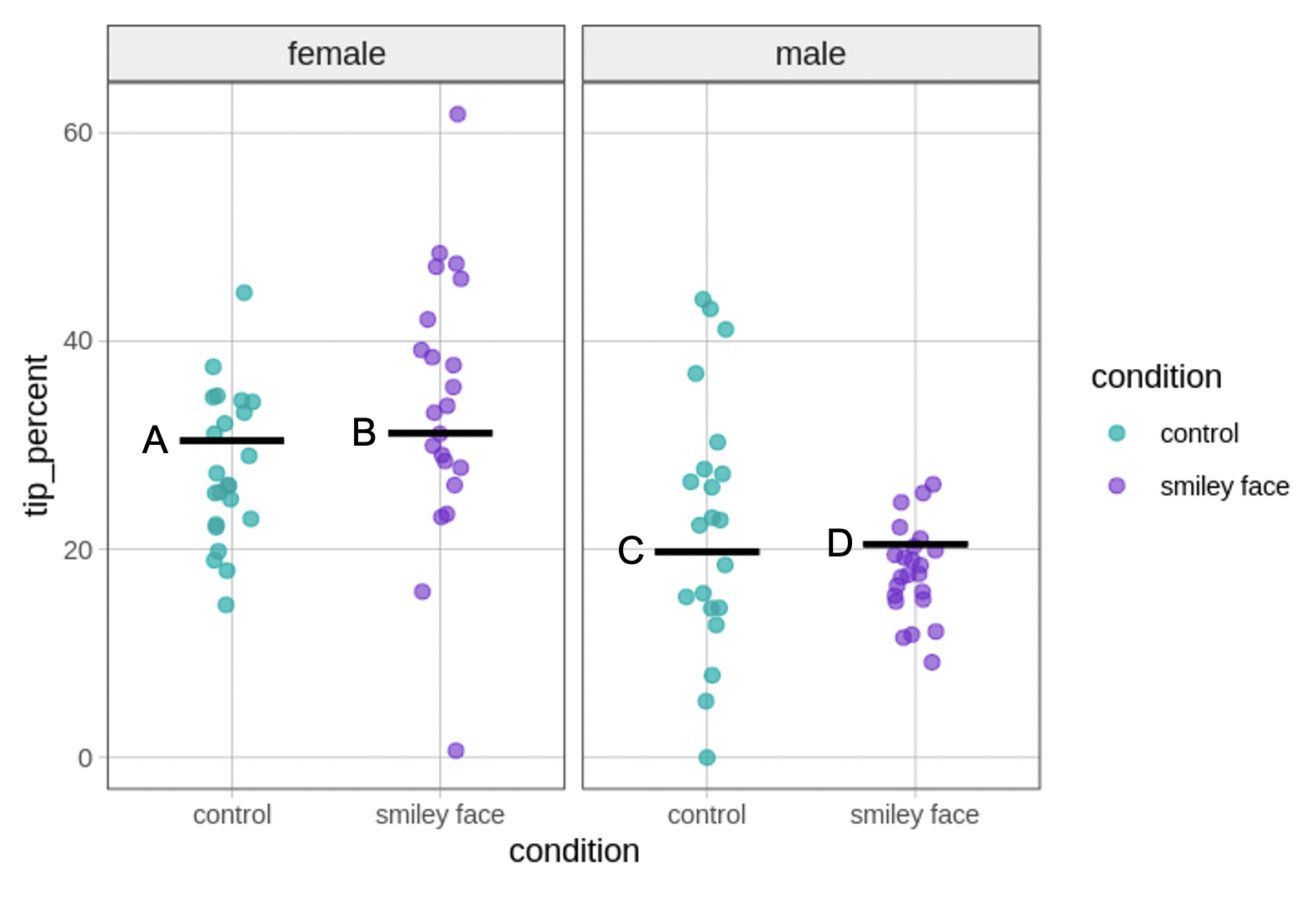## Course Outline

• segmentGetting Started (Don't Skip This Part)
• segmentStatistics and Data Science: A Modeling Approach
• segmentPART I: EXPLORING VARIATION
• segmentChapter 1 - Welcome to Statistics: A Modeling Approach
• segmentChapter 2 - Understanding Data
• segmentChapter 3 - Examining Distributions
• segmentChapter 4 - Explaining Variation
• segmentPART II: MODELING VARIATION
• segmentChapter 5 - A Simple Model
• segmentChapter 6 - Quantifying Error
• segmentChapter 7 - Adding an Explanatory Variable to the Model
• segmentChapter 8 - Models with a Quantitative Explanatory Variable
• segmentPART III: EVALUATING MODELS
• segmentChapter 9 - The Logic of Inference
• segmentChapter 10 - Model Comparison with F
• segmentChapter 11 - Parameter Estimation and Confidence Intervals
• segmentPART IV: MULTIVARIATE MODELS
• segmentChapter 12 - Introduction to Multivariate Models
• segmentChapter 13 - Multivariate Model Comparisons
• segmentFinishing Up (Don't Skip This Part!)
• segmentResources

### list Statistics and Data Science: A Modeling Approach

Book
• College / Advanced Statistics and Data Science (ABCD)
• College / Statistics and Data Science (ABC)
• High School / Advanced Statistics and Data Science I (ABC)
• High School / Statistics and Data Science I (AB)
• High School / Statistics and Data Science II (XCD)

## 13.8 Models with Multiple Categorical Predictors

Now that you have learned to add on new predictor variables, you can go out there and make all kinds of multivariate models. We have mostly focused on a model that has two predictors but you can now make models with 3, 4 or 10 explanatory variables! Just know that each additional parameter you estimate is going to cost you degrees of freedom.

The multivariate model we have focused on so far has one categorical predictor (Neighborhood) and one quantitative predictor (HomeSizeK). But the General Linear Model does not limit us. We could build models with multiple categorical predictors, multiple quantitative predictors, or both. On this page we’ll fit a model with two categorical predictors, on the next page, one with two quantitative predictors.

### Investigating the Effects of Smiley Face and Gender on Tips

In earlier chapters we analyzed data from the tipping experiment, in which tables of diners in a restaurant were randomly assigned to one of two conditions: in the Smiley Face condition the server drew a smiley face on the check at the end of the meal; in the other condition they did not. We were interested in whether tables in the smiley face condition tipped more than tables with no smiley face.

Here we are going to analyze data from a follow-up study with two predictor variables. As before, tables were randomly assigned to get a smiley face or not on the check. But this time, tables within each condition were also randomly assigned to get either a female or male server. This kind of experiment is sometimes called a “two-by-two factorial design.” There are two predictor variables (condition and gender), and each predictor has two levels.

The data are saved in a data frame called tip_exp. Here’s some code we ran to see what’s in the data, and to see how many tables were in each of the four different cells of the two-by-two design.

str(tip_exp)
tally(gender ~ condition, data=tip_exp)
'data.frame':   89 obs. of  3 variables:
$gender : chr "male" "male" "male" "male" ...$ condition  : chr  "control" "control" "control" "control" ...
\$ tip_percent: num  14.3 18.5 41.1 22.3 23 …

condition
gender   control smiley face
female      23          22
male        21          23

We can see that there were 89 tables included in the study. We also can see that there are approximately the same number of tables in each of the four groups (from 21 to 23). This is a result of the experimental design. But it’s important because it means we are likely to have very little redundancy between the two predictor variables when we create a model of tip_percent.

### Explore Variation in tip_percent

We can visualize this hypothesis with a number of different plots (e.g., histograms, boxplots, jitter plots, scatter plots). We’ve selected a jitter plot, faceted by gender, using color to highlight the comparison between tables that did versus did not get the smiley face.

gf_jitter(tip_percent ~ condition, color = ~condition, data = tip_exp, width = .2) %>%
gf_facet_grid(. ~ gender)### Model Variation in tip_percent

In the code window below, use lm() to fit the tip percent = condition + gender model to the data.

require(coursekata) # delete when coursekata-r updated set.seed(123) # male - control m1 <- 21.41 sd1 <- 12.64 n1 <- 21 # female - control m2 <- 27.78 sd2 <- 7.77 n2 <- 23 # male - smiley m3 <- 17.78 sd3 <- 5.57 n3 <- 23 # female - smiley m4 <- 33.04 sd4 <- 14.02 n4 <- 22 gender <- factor(c(rep("male", n1),rep("female", n2),rep("male", n3),rep("female", n4))) condition <- factor(c(rep("control", n1),rep("control", n2),rep("smiley face", n3),rep("smiley face", n4))) tip_percent <- round(c(rnorm(n1, m1, sd1), rnorm(n2, m2, sd2), rnorm(n3, m3, sd3), rnorm(n4, m4, sd4)), 2) tip_percent <- 0 tip_exp <- data.frame(gender, condition, tip_percent) # find the best fitting parameter estimates  lm(tip_percent ~ condition + gender, data = tip_exp) # temporary SCT ex() %>% check_error()
CK Code: D2_Code_Categorical_01
Call:
lm(formula = tip_percent ~ condition + gender, data = tip_exp)

Coefficients:
(Intercept)  conditionsmiley face            gendermale
30.4264                0.7395              -10.6730 

If we save our multivariate model as multi_model, we can use gf_model(multi_model) to put it on our jitter plot. Try it in the code window below.

require(coursekata) # delete when coursekata-r updated set.seed(123) # male - control m1 <- 21.41 sd1 <- 12.64 n1 <- 21 # female - control m2 <- 27.78 sd2 <- 7.77 n2 <- 23 # male - smiley m3 <- 17.78 sd3 <- 5.57 n3 <- 23 # female - smiley m4 <- 33.04 sd4 <- 14.02 n4 <- 22 gender <- factor(c(rep("male", n1),rep("female", n2),rep("male", n3),rep("female", n4))) condition <- factor(c(rep("control", n1),rep("control", n2),rep("smiley face", n3),rep("smiley face", n4))) tip_percent <- round(c(rnorm(n1, m1, sd1), rnorm(n2, m2, sd2), rnorm(n3, m3, sd3), rnorm(n4, m4, sd4)), 2) tip_percent <- 0 tip_exp <- data.frame(gender, condition, tip_percent) # write code to save this model as multi_model lm(formula = tip_percent ~ condition + gender, data = tip_exp) # this makes a faceted jitter plot of our data # write code to overlay the multi_model onto it gf_jitter(tip_percent ~ condition, color = ~condition, data = tip_exp, width = .1) %>% gf_facet_grid(. ~ gender) # will eventually work multi_model <- lm(formula = tip_percent ~ condition + gender, data = tip_exp) gf_jitter(tip_percent ~ condition, color = ~condition, data = tip_exp, width = .1) %>% gf_facet_grid(. ~ gender) %>% gf_model(multi_model) # temporary SCT ex() %>% check_error()
CK Code: D2_Code_Categorical_02We have put some letters on our version of the plot to make it easier to refer to. Let’s take a moment to connect the parameter estimates in our model to the model predictions shown as black lines (labeled A, B, C, D) in the visualization.

The $$b_1$$ estimate (0.74) represents the amount added to the prediction of tip_percent for tables in the smiley face condition controlling for gender. The $$b_2$$ estimate (-10.67) represents the amount added to the prediction of tip_percent for tables with a male server controlling for condition.

One thing to notice about this model is that it assumes that the difference between female and male servers, for example, which is -10.6730, is the same for tables in the control condition as for those in the smiley face condition. Likewise, the effect of condition is assumed to be the same, regardless of whether the servers are female or male. We will relax this assumption in the next chapter, when we turn our attention to models with interactions.

We can summarize the connections between the parameter estimates and the plot we made by labeling the lines with their corresponding parameter estimates in the figure below.